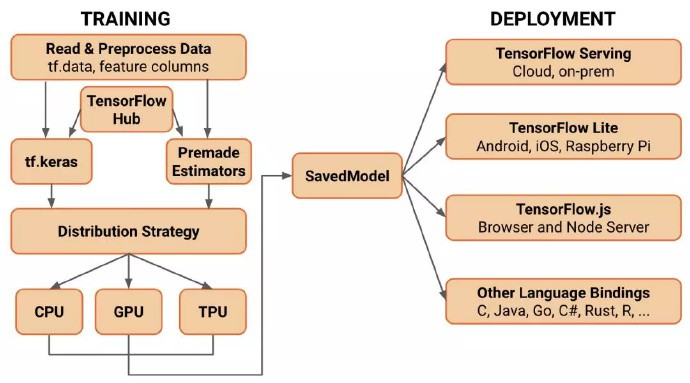TensorFlow 已经发展为世界上最受欢迎和被广泛采用的机器学习平台之一，我们衷心感谢一直以来支持我们的各界的开发者和他们的贡献：

2018 年 11 月，TensorFlow 迎来了它的 3 岁生日，我们回顾了几年来它增加的功能，进而对另一个重要里程碑 TensorFlow 2.0 感到兴奋 ！

TensorFlow 2.0 将专注于 简单性易用性，具有以下更新：

• 使用 Keras 和 eager execution，轻松构建模型
• 在任意平台上实现生产环境的稳健模型部署
• 为研究提供强大的实验工具
• 通过清理废弃的 API 和减少重复来简化 API## 1. 标准化 Keras：TensorFlow 2.0 高级 API 指南

Keras 是一个颇受欢迎的高级 API，专用于构建和训练深度学习模型. 此 API 可用于快速原型设计、顶尖研究与实际生产. TensorFlow 现已支持 Keras，但在 2.0 版中，我们正致力将 Keras 更紧密地集成至 TensorFlow 平台的其余组件.

Keras 具备以下几项关键优势：

• 人性化：Keras 界面简单、一致，已针对常见用例进行优化. Keras 能够就用户错误提供清晰且可处理的反馈，不仅便于用户了解错误消息，而且通常还能提供实用建议.
• 模块化与可组合性：Keras 模型由可配置的构建基块相连组合而成，几乎不存在任何限制. Keras 的部分组件可重复利用，用户将无需采用甚至了解框架提供的全部内容. 例如，无需通过 Keras 模型开展训练便可使用层或优化器.
• 易于扩展：可以通过编写自定义构建基块来展现新的研究思路，包括构建新层、损失函数等.
• 初学者和专家通用：深度学习开发者背景不同、经验水平各异，而无论您是刚刚入门，还是拥有多年经验，Keras 均可提供切合需求的 API.

### 1.1. 常见问题解答

#### 1.1.1. Keras 是否为一个独立的库？

Keras 的参考实现一直作为独立的开放源代码项目进行维护，具体可于此网站找到：www.keras.io. 该项目独立于 TensorFlow，并拥有一个活跃的贡献者与用户社区.

TensorFlow 包括 Keras API 的完整实现（位于 tf.keras 模块），且此 API 具备针对 TensorFlow 的增强功能.

#### 1.1.2. Keras 只是 TensorFlow 或其他库的包装器吗？

Keras 是一种用于定义和训练机器学习模型的 API 标准.

Keras 与特定实现无关：Keras API 可用于 TensorFlow、MXNet、TypeScript、JavaScript、CNTK、Theano、PlaidML、Scala、CoreML 和其他库的实现.

#### 1.1.3. TensorFlow 内置的 Keras 版本与 keras.io 提供的版本有何不同？

TensorFlow 包括 Keras API 的具体实现（位于 tf.keras 模块），且此 API 具备针对 TensorFlow 的增强功能. 这些功能包括：支持 Eager Execution 以便进行直观调试和快速迭代支持 TensorFlow SavedModel 模型交换格式，以及为分布式训练（包括 TPU 上的训练）提供集成支持.

tf.keras 紧密集成于 TensorFlow 生态系统，同时也支持：

• tf.data：可构建高性能输入管道. 若您有意尝试，则可使用 NumPy 格式的数据训练模型，或使用 tf.data 来扩大规模和提升性能.
• 分布策略：该策略用于在各类计算配置中开展分布式训练，包括遍布于众多机器上的 GPU 和 TPU.
• 导出模型：利用 tf.keras API 创建的模型能够以 TensorFlow SavedModel 格式进行序列化，并能借助 TensorFlow Serving 或通过其他语言绑定（Java、Go、Rust、C# 等）提供给用户使用.
• 导出的模型可通过 TensorFlow Lite 部署至移动和嵌入式设备，同时也适用于 TensorFlow.js（注：也可使用同样常见的 Keras API 直接在 JavaScript 中开发模型）.
• 特征列：用于有效表示及分类结构化数据.
• 此外，tf.keras 还可支持其他正在开发的内容.

### 1.2. tf.keras 的安装使用

tf.keras 内附于 TensorFlow 中. 您无需单独安装 Keras.

pip install tensorflow
pip install tensorflow-gpu

tf.keras 的使用：

import tensorflow as tf

Dense = tf.keras.layers.Dense

### 1.3. Sequential API

import tensorflow as tf
mnist = tf.keras.datasets.mnist

(x_train, y_train), (x_test, y_test) = mnist.load_data()
x_train, x_test = x_train / 255.0, x_test / 255.0

model = tf.keras.models.Sequential([
tf.keras.layers.Flatten(),
tf.keras.layers.Dense(512, activation=tf.nn.relu),
tf.keras.layers.Dropout(0.2),
tf.keras.layers.Dense(10, activation=tf.nn.softmax)
])

loss='sparse_categorical_crossentropy',
metrics=['accuracy'])
model.fit(x_train, y_train, epochs=5)
model.evaluate(x_test, y_test)

model = tf.keras.Sequential()
Such a model can then be compiled and trained in a few lines:
loss=’sparse_categorical_crossentropy’,
metrics=[‘accuracy’])model.fit(x_train, y_train, epochs=5)
model.evaluate(x_test, y_test)

### 1.4. Functional API

inputs = tf.keras.Input(shape=(32,))
# A layer instance is callable on a tensor, and returns a tensor.
x = layers.Dense(64, activation=’relu’)(inputs)
x = layers.Dense(64, activation=’relu’)(x)
predictions = layers.Dense(10, activation=’softmax’)(x)
# Instantiate the model given inputs and outputs.
model = tf.keras.Model(inputs=inputs, outputs=predictions)

### 1.5. Model Subclassing API

class MyModel(tf.keras.Model):
def __init__(self):
super(MyModel, self).__init__()
self.dense_1 = layers.Dense(32, activation=’relu’)
self.dense_2 = layers.Dense(num_classes, activation=’sigmoid’)

def call(self, inputs):
# Define your forward pass here,
# using layers you previously defined in __init__
x = self.dense_1(inputs)
return self.dense_2(x)

model = MyModel()

logits = model(images, training=True)
loss_value = loss(logits, labels)

optimizer.apply_gradients(zip(grads, model.variables))

### 1.6. 如果以上三种模式均不适用，该怎么办？

• 单独使用 Keras 模型定义中的 tf.keras.layers，然后自行编写梯度和训练代码. 还可单独或独立使用 tf.keras.optimizers、tf.keras.initializers、tf.keras.losses 或 tf.keras.metrics.
• 此外，也可完全忽略 tf.keras，并使用较低层级的 TensorFlow、Python 和 AutoGraph 获取期望结果.

，tf.layers 中的非面向对象层将被弃用，且 tf.contrib.*（包括 tf.contrib.slim 和 tf.contrib.learn 等高级 API）将无法在 TensorFlow 2.0 中使用.

### 1.7. Estimator 有何变化？

Estimator 在 Google 内部以及更广泛的 TensorFlow 社区中应用甚广. 我们已将数种模型打包为预创建的 Estimator，包括线性分类器、DNN 分类器、DNN 线性组合分类器（亦即广度与深度模型）和梯度提升树. 这些模型已投入生产并得到广泛部署；基于此，TensorFlow 2.0 中将会添加 Estimator API（包括预创建的 Estimator）.

## 2. 简单的模型构建

Keras API 将成为 TensorFlow 中构建和训练模型的核心高级 API.

Keras API 使得使用 TensorFlow 开启项目变得简单. 重要的是，Keras 提供了几个模型构建 API ( Sequential, Functional, 和 Subclassing )，因此可以选择适合的抽象级别. TensorFlow 的实现包含多项增强功能，包括即时迭代和直观调试，以及 tf.data，用于构建可扩展的输入管道.

 - 使用 tf.data 加载数据. 使用输入管道读取训练数据，用 tf.data 创建的输入线程读取训练数据. 使用 tf.feature_column 描述特征，例如嵌套和特征交叉. 还支持从内存数据（例如 NumPy）中方便地输入

 - 使用 tf. Keras 或 Premade Estimators 构建、训练和验证模型. Keras 与 TensorFlow 的其余部分紧密集成，因此可以随时访问 TensorFlow 的功能. 一组标准的打包模型 ( 例如，线性或逻辑回归、梯度增强树、随机森林 ) 也可以直接使用 ( 利用 tf.estimator API 实现 ). 如果不想从头开始训练一个模型，很快就能通过 TensorFlow Hub 的模块利用迁移学习来训练 Keras 或 Estimator 模型

 - 使用 eager execution 运行和调试，然后在图形上使用 tf.function. TensorFlow 2.0 在默认情况下以 eager execution 方式运行，以便于使用和顺利调试. 此外，tf.function annotation 透明地将 Python 程序转换成 TensorFlow 图. 这个过程保留了 1.x TensorFlow 基于图执行的所有优点：性能优化、远程执行以及轻松序列化、导出和部署的能力，同时增加了使用简单的 Python 表达程序的灵活性和易用性

 - 使用分布策略进行分布式训练. 对于大型机器学习训练任务来讲，Distribution Strategy API 使得在不更改模型定义的情况下，在不同硬件配置上分布和训练模型变得很容易. 由于 TensorFlow 提供了对 CPU、GPU 和 TPU 等一系列硬件加速器的支持，因此可以将训练工作负载分布到单节点 / 多加速器以及多节点 / 多加速器配置，包括 TPU Pods. 虽然此 API 支持各种集群配置，但还提供了在本地或云环境中部署 Kubernetes 集群训练的模板

 - 导出到 SavedModel. TensorFlow 将在 SavedModel 上标准化，作为 TensorFlowServing、TensorFlow Lite、TensorFlow.js、TensorFlow Hub 等的交换格式

TensorFlow 指南详细信息

## 3. 在任何平台上的生产环境中进行稳健的模型部署

TensorFlow 始终为生产提供了直接途径. 无论是在服务器、边缘设备或者 web上，无论您使用哪种语言或平台，TensorFlow 都可以轻松地训练和部署模型. 在 TensorFlow 2.0 中，我们通过标准化交换格式和调整 API 来提高平台和组件之间的兼容性和一致性.

• TensorFlow Serving：TensorFlow 库允许模型通过 HTTP / REST 或 gRPC / 协议缓冲区提供服务
• TensorFlow Lite：TensorFlow 针对移动和嵌入式设备的轻量级解决方案提供了在 Android、iOS 和 Raspberry Pi 和 Edge tpu 等嵌入式系统上部署模型的能力
• TensorFlow.js：支持在 JavaScript 环境中部署模型，例如通过 Node.js 在 web 浏览器或服务器端部署模型. TensorFlow.js 还支持用 JavaScript 定义模型，并使用类似于 keras 的 API 直接在 web 浏览器中进行训练

TensorFlow 还支持其他语言 ( 一些由更广泛的社区维护 )，包括 C, Java, Go, C#, Rust, Julia, R 等.

## 4. 为研究提供强大的实验工具

TensorFlow 使从概念到代码、从模型到发布的新思想变得容易. TensorFlow 2.0 集成了许多功能，可以在不牺牲速度或性能的情况下定义和训练最新模型：

• Keras Functional API 和 Model Subclassing API：允许创建复杂的拓扑，包括使用剩余层、自定义的多输入 / 输出模型和强制写入的正向传递
• 为了获得更大的灵活性和更好的控制，低级别 TensorFlow API 始终可用，并与高级别抽象结合在一起，以实现完全可定制的逻辑

TensorFlow 2.0 带来了一些新功能，允许研究人员和高级用户使用丰富的扩展 ( 如 Ragged Tensors, TensorFlow Probability, Tensor2Tensor 等) 进行实验.

## 5. TensorFlow 1.x 和 2.0 的区别

• 删除 queue runner 以支持 tf.data
• 删除图形集合
• 改变变量的处理方式
• 符号的移动和重命名

https://github.com/tensorflow/community/pull/37

## 6. 兼容性和连续性

https://github.com/tensorflow/docs/blob/master/site/en/r2/guide/effective_tf2.md

## 7. TensorFlow 2.0 的时间表

TensorFlow 2.0 预览版将于今年年初发布.

Last modification：January 16th, 2019 at 02:53 pm